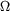# LRC circuit and phase angle

• ooohffff

## Homework Statement

A 35 mH inductor with 1.0resistance is connected in series to a 20 µF capacitor and a 60 Hz, 40-V (rms) source. Calculate the phase angle.

## Homework Equations

tan φ = (XL - XC) / R

## The Attempt at a Solution

Solving for φ:

φ = tan -1 [(XL - XC) / R]

XL = 2πfL = 13.194 Ω
XC = 1/(2πfC) = 132.63 Ω
R=1Ω

Plugging those values in I get
Φ = -89.5° = 270.48°

I submitted the 270.48 but it's wrong?

What you've found is the phase angle of the impedance. You want the phase angle of the current. What law relates the current to the voltage and impedance?

What you've found is the phase angle of the impedance. You want the phase angle of the current. What law relates the current to the voltage and impedance?

Ohm's law I=V/Z ?

Ohm's law I=V/Z ?
Yup. What will be the current's angle if your Z's angle is -89.5° ?

Yup. What will be the current's angle if your Z's angle is -89.5° ?
Would it be tan φ = (VL - VC) / Z ? If not then I'm thoroughly confused.

No. The only voltage of consequence here is the supply voltage. If this was a DC circuit with resistors you'd write I = V/R. In this AC circuit you write I = V/Z.

V is the source voltage that also serves as the reference for the phase angle. As such its phase angle is 0°. Your Z has a phase angle of -89.5°. So what's the phase angle of V/Z? (How do you handle angles when you do a division)?

No. The only voltage of consequence here is the supply voltage. If this was a DC circuit with resistors you'd write I = V/R. In this AC circuit you write I = V/Z.

V is the source voltage that also serves as the reference for the phase angle. As such its phase angle is 0°. Your Z has a phase angle of -89.5°. So what's the phase angle of V/Z? (How do you handle angles when you do a division)?

Would you break it into components like:
I = (40/(Zcosφ)) i + (40/(Zsinφ)) j

and then find the angle of I?

No need. You have the angles already. There's a simple rule for dividing two complex numbers when you know the angles. What's the rule?

No need. You have the angles already. There's a simple rule for dividing two complex numbers when you know the angles. What's the rule?

I just derived this because I don't think I know what you're talking about or I'm misguided, but I got tan-1 (cotφ)

No, you're getting way too complicated. When you divide two numbers in complex polar form you simply subtract the angle of the denominator from the angle of the numerator:

##\frac{a ∠ θ}{b ∠ φ} = \left(\frac{a}{b}\right) ∠ (θ - φ)##

No, you're getting way too complicated. When you divide two numbers in complex polar form you simply subtract the angle of the denominator from the angle of the numerator:

##\frac{a ∠ θ}{b ∠ φ} = \left(\frac{a}{b}\right) ∠ (θ - φ)##
But then the resulting angle would be at 89.5°?

But then the resulting angle would be at 89.5°?
I've tried that before initially but that angle was also incorrect.

But then the resulting angle would be at 89.5°?
Yes. That should be the phase angle of the current with respect to the voltage.

I've tried that before initially but that angle was also incorrect.
You tried +89.5° and it was considered incorrect? Perhaps they're being picky about significant figures?

Yes. That should be the phase angle of the current with respect to the voltage.

You tried +89.5° and it was considered incorrect? Perhaps they're being picky about significant figures?

Yup. That was the first one I tried with the equation: φ = cos -1 (R/Z) = 89.52028539°

Yes, maybe I should try with more sig figs.

How many significant figures does the given data suggest?

How many significant figures does the given data suggest?

Ah I figured out the problem! My original answer in this post -89.5° was correct. The mistake I made was that I should not have converted it to 270.48°, since technically φ should be negative since XC > XL, and you should generally take the smaller angle of the angles between two vectors.

What was the exact phrasing of the question as you received it? Generally "phase angle", unless qualified, refers to the phase angle of the current with respect to the voltage. Were they only looking for the phase angle of the impedance?

What was the exact phrasing of the question as you received it? Generally "phase angle", unless qualified, refers to the phase angle of the current with respect to the voltage. Were they only looking for the phase angle of the impedance?

That is the exact phrasing of the question. That phase angle, according to the formula that I used, should be the angle between voltage and current, not impedance and current.

tanΦ = (VL-VC )/ VR = (XL - XC)/ R

Yes, it yields the angle associate with the impedance which is also the phase angle of the current with respect to the voltage.

But as I mentioned previously, unless otherwise specified, generally when one talks about phase angle one is referring to the phase angle of the current with respect to that of the voltage, not the voltage with respect to the current. Basically, I'm not very happy with the problem as it is presented. But if you reached the answer that they're looking for, not much more can be said